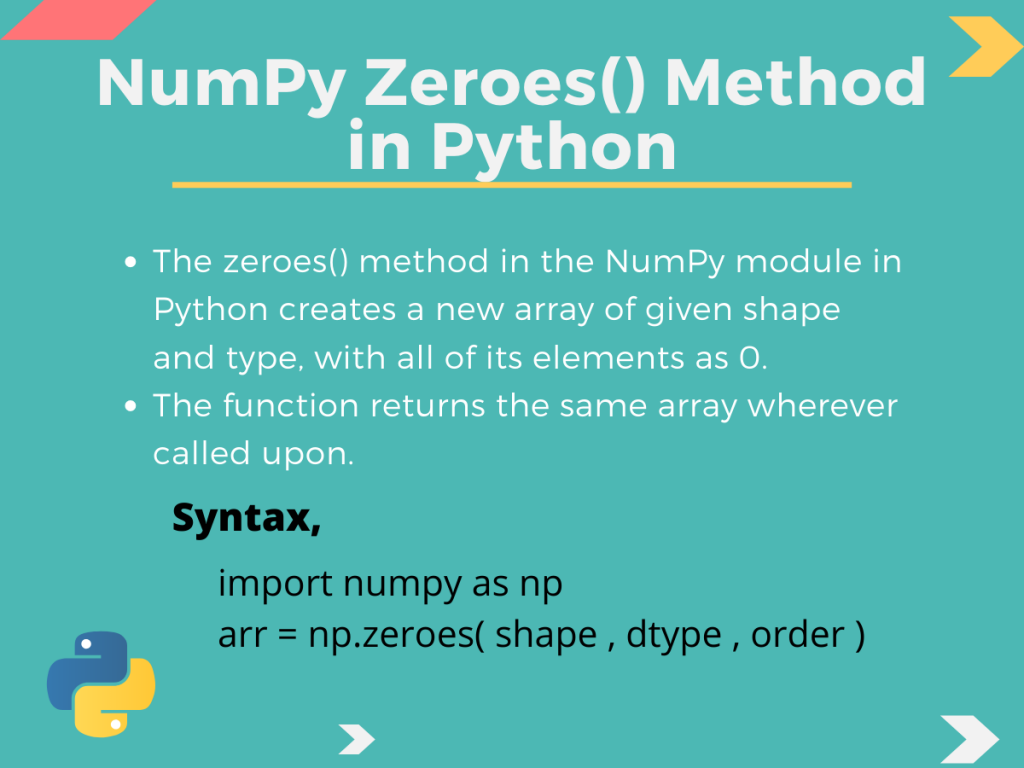# NumPy zeros() Method in Python## Introduction

NumPy is a vastly implemented module in Python. Today we’re going to learn the Numpy `zeros()` method is one of the defined methods in NumPy.

So, let us get right into it!

## The Numpy zeros() method in Python

The Numpy `zeros()` method in Python creates a new array of the specified shape and type, with all of its elements initialized to 0. The function returns the same array wherever called upon.

The basic syntax of the `zeros()` method can be given by,

```import numpy as np

arr = np.zeros( shape , dtype , order )
```

Here,

• arr stores the resultant array returned by the `zeros()` method,
• The `shape` is an integer of sequence, which decides the size as well as the shape of the array. When the shape is provided as an integer number, the array generated would be a 1D array. Whereas, if we give an integer tuple with different integer values, we are going to get a multi-dimensional array,
• `dtype` or data type specifies the data type of the array elements. By default, it is set to float.
• `Order` decides whether the array is stored according to row-major(C) or column-major(F) pattern/order in the memory location.

## How to use Numpy zeros() in Python?

Now that we know what the Nump `zeros()` method in Python does, let us learn the usage.

## 1D array using Numpy zeros()

We can create one-dimensional arrays in Python with all of its elements being zero(0) using this method. Let us take an example into consideration for better understanding.

```import numpy as np

arr1 = np.zeros(5)
print("The created array is: ",arr1) #generated array
print("It is of type:",type(arr1)) #type of array
```

Output:

Here, in the code above,

• arr1 is the new array created. As we can see, we have just passed ‘5‘ to the Numpy `zeros()` function, with no data type and order.
• By default, the values of the `dtype` and order is considered as float and ‘C‘ respectively. That means the generated array is going to have float type elements and be stored in a row-major form.
• Finally, when we print out the array, we get a 1D array with all of its float elements having a value 0. And the type() of arr1 tells us that it is a member of the `ndarray` class.

## Array with Different Data Types Using Numpy zeros()

So, we created an array with default type float. What if we need an array that has integer values or elements? We can easily do that by specifying the `dtype` parameter as our desired type. Let us see how

```import numpy as np

int_array = np.zeros(4, dtype=int)  #data type set as int
print("Array: ",int_array) #the final int array
print("Datatype: ", int_array.dtype) # the data types
```

Output:

Here,

• int_array is the new array created by using the `zeros()` method.
• As we can see from the output, we create an array of size 4 as integer type. Again the data type of the elements is given as int32.(integer).

## Multi-Dimensional Array Using Numpy zeros()

We created a 1D array previously in this tutorial, so what about multi-dimensional ones? Let us try to create a 2D array to illustrate how we can create multi-dimensional arrays having elements as 0.

```import numpy as np

arr2 = np.zeros((3, 3), int) #2D integer array
print("2D array created is: ",arr2)
print("Type of the array: ",type(arr2)) #type of the array
```

Output:

In the code above:

• We pass a tuple of integers to the Numpy `zeros()` method instead of a single one. This allows us to create a multi-dimensional array of int type(specified).
• As we can see from the output, we get a 2D array with all of the elements as 0.

## Arrays of Heterogeneous Data Type Using Numpy zeros()

We can also create arrays with heterogeneous data type using the `zeros()` function in Python. We just need to pass a tuple containing the required information.

```import numpy as np

# creating array with heterogeneous data types
arr = np.zeros((2,2), dtype=[('x', 'int'), ('y', 'float')])
print("The array created:",arr)
print("Type of the array: ",type(arr)) #type of the array
print("Datatype: ", arr.dtype) # the data types
```

Output:Heterogeneous Data Type Array Using Zeros

Here,

• We create a two-dimensional array by passing shape as a tuple (2,2),
• In the case of the data type, we use a tuple, specifying both integer and float types,
• This results in the creation of a 2D array having tuple elements. Each tuple has two data, one of `int` type and the other of `float `type,
• Lastly, we print the data type of the member elements as shown in the out.

## Conclusion

So in this tutorial, we discussed the Numpy `zeros()` method in Python. Hope it was understood well. For any questions feel free to use the comments below.